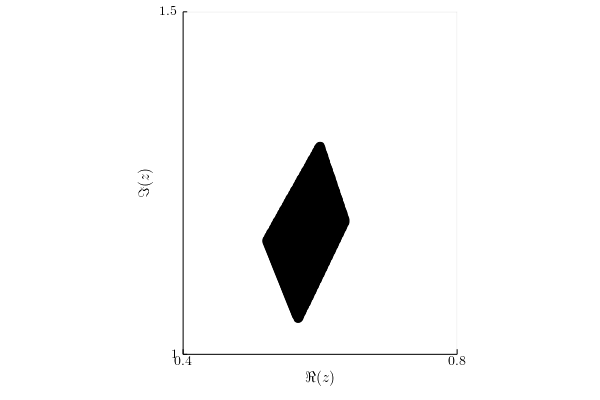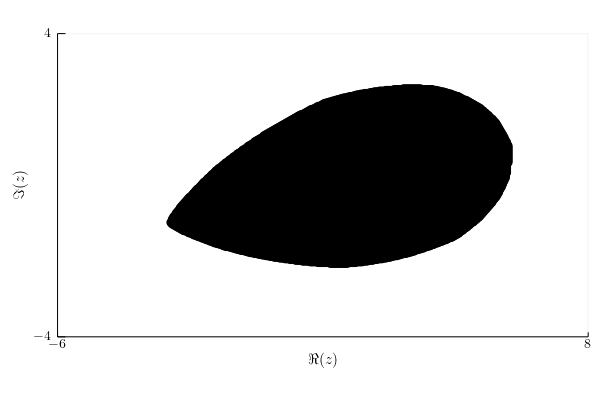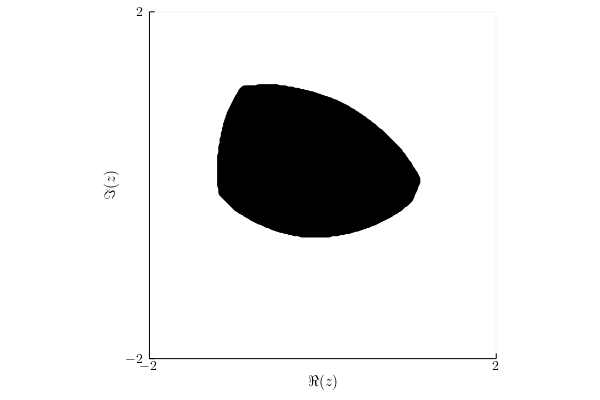# Higher rank numerical range of rectangular matrices

The notion of higher rank numerical ranges of rectangular matrices were first introduced by Mohsen Zahraei and Gholamreza Aghamollaei in their work . They showed that, the proposed definition is a generalization of well-known higher numerical range of square matrices and numerical range of rectangular matrices.

Let $M,N$ be positive integers, that satisfy $M \geq N$. For every $k \in \{1,\ldots,N\}$ define the following set: $\mathcal{X}= \left\{ (X,Y): X \mbox{ is } N \times (N-k+1) \mbox{ isometry matrix }, Y=\left[ \begin{array}{c|c} X & 0\ \hline 0 & U \end{array} \right],U \in \mathcal{U}_{M-N} \right\}.$

## Definition

Let $A$ and $B$ be $N \times M$ matrices and let $\| \|$be any matrix norm. The rank-k numerical range of$A$ with respect to $B$ is defined as ${k,\| \|}(A; B) = \{ : \|X^(A- B)Y \| |- |, , (X,Y) \}.$ If $N \geq M$, then we use redifned set $\mathcal{X}$, namely $\mathcal{X}= \left\{ (X,Y): Y \mbox{ is } M \times (M-k+1) \mbox{ isometry matrix }, X=\left[ \begin{array}{c|c} Y & 0\ \hline 0 & U \end{array} \right],U \in \mathcal{U}_{N-M} \right\}$ in the previous definition. First of all, if $k=1$ and the norm $\|\cdot \|$ is unitarily invariant, then $*{1,\|\|}(A;B)=w_{\| \|}(A; B),$ which is the numerical range of rectangular matrices. Moreover, if $A$ is $N \times N$ square matrices, then for $\| \|=\| \|$ we have $*{k,\| \|}(A;\1_N)=_{k}(A),$ which is the higher rank numerical range.

## Properties

The most important one is the representation form: $*{k,\|\|}(A;B)= *{(X,Y) } w_{\| \|}(X^A Y; X^B Y).$ This leads to conclusion, that as requested, $\Lambda_{k,\|\cdot \|}(A;B)$ is a compact and convex set in $\mathbb{C}$.

Assume now $N \geq M$. The casual numerical range cointains convex hull of eigenvalues, so it would be delightfull if it was true for introduced numerical range $\Lambda_{k,\|\cdot \|}(A;B)$. Spectrum of rectangular matrix $A$ with respect to $B$ is defined as $(A;B)=\{ : (A-B) 1\}.$ For more details of this set see . The proposed generalization of $\sigma(A;B)$ is $k-$spectrum of $A$ with respect to $B$ defined and denoted by $_k(A;B)=\{: (X^(A - B) Y) k, (X,Y) \},$ for $k \leq [(M+1)/2]$. Under this assumptions, if $\| \cdot \|$ is induced matrix norm and for every unit vector $z$ and for all $(X,Y) \in \mathcal{X}$ we have $\| X^\dagger B Y z \| \geq 1$, then $*k(A;B) *{k,\| \|} (A;B).$

## Example 1

Diagonal matrix $A=\begin{pmatrix} 1 & 0 & 0 & 0\ 0 & 1+2\ii & 0 & 0\ 0 & 0 & 3\ii & 0\ 0 & 0 & 0 & 0 \end{pmatrix}$ with respect to matrix $B =\14$ with $||\cdot|| = ||\cdot||\infty$. Then, $\Lambda_{k,||\cdot||}(A;B) = \Lambda_k(A)$ is $k$–numerical range of $A$. In this case, $k=2$.## Example 2

Matrix $A=\begin{pmatrix} 6+\ii & 0.4 & 0 & -0.1\ 0.1 & 1-3\ii & -0.3\ii & 0\ 0 & 0 & 0.5 & 0 \end{pmatrix}$ with respect to matrix $B=\begin{pmatrix} 1.2 & 0 & 0 & 0\ 0 & \ii & 0 & 0\ 0 & 0 & -1 & 0 \end{pmatrix}$ with $|||| = ||||_$and$k=1$. Then, $\Lambda_{1,||\cdot||}(A;B) = w_{\| \cdot \|}(A; B)$.## Example 3

Two random Ginibre matrices $A$ and $B$ of dimension $5 \times 4$ with $||\cdot|| = ||\cdot||_\infty$ and $k=3$.1. M. Zahraei, G. Aghamollaei, and others, “Higher rank numerical ranges of rectangular matrices,” Annals of Functional Analysis, vol. 6, no. 2, pp. 133–142, 2015, [Online]. Available at: https://projecteuclid.org/euclid.afa/1418997772.
2. C. Chorianopoulos, S. Karanasios, and P. Psarrakos, “A definition of numerical range of rectangular matrices,” Linear and Multilinear Algebra, vol. 57, no. 5, pp. 459–475, 2009, [Online]. Available at: https://www.tandfonline.com/doi/abs/10.1080/03081080802466365.